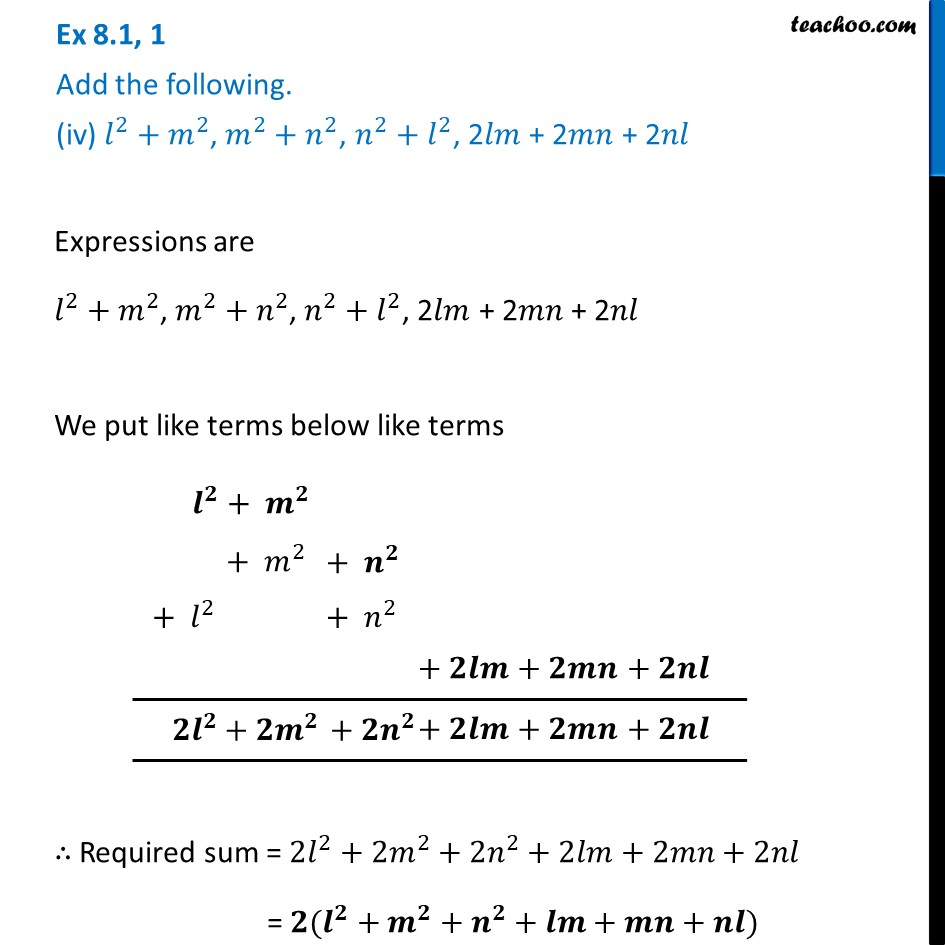Ex 8.1

Chapter 8 Class 8 Algebraic Expressions and Identities
Serial order wiseLearn in your speed, with individual attention - Teachoo Maths 1-on-1 Class

### Transcript

Ex 8.1, 1 Add the following. (iv) 𝑙^2 〖+ 𝑚〗^2, 𝑚^2 〖+ 𝑛〗^2, 𝑛^2 〖+ 𝑙〗^2, 2𝑙𝑚 + 2𝑚𝑛 + 2𝑛𝑙Expressions are 𝑙^2 〖+ 𝑚〗^2, 𝑚^2 〖+ 𝑛〗^2, 𝑛^2 〖+ 𝑙〗^2, 2𝑙𝑚 + 2𝑚𝑛 + 2𝑛𝑙 We put like terms below like terms ∴ Required sum = 2𝑙^2+2𝑚^2+2𝑛^2+2𝑙𝑚+2𝑚𝑛+2𝑛𝑙 = 𝟐(𝒍^𝟐+𝒎^𝟐+𝒏^𝟐+𝒍𝒎+𝒎𝒏+𝒏𝒍)